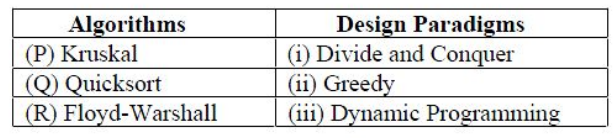# GATE | GATE-CS-2017 (Set 1) | Question 29

• Last Updated : 28 Jun, 2021

Consider the following tableMatch the algorithm to design paradigms they are based on:
(A) P-(ii), Q-(iii), R-(i)
(B) P-(iii), Q-(i), R-(ii)
(C) P-(ii), Q-(i), R-(iii)
(D) P-(i), Q-(ii), R-(iii)